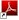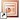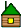## Статистика

Kyle Cranmer
Practical Statistics for Particle Physicists
Статистика для физики частиц

Glen Cowan (RHUL)
Practical Statistics for Particle Physicists
Практическая статистика для физики частиц

Helge Voss (Physik-Institut)
Statistics in HEP
Статистика на БАК

Harrison Prosper (Florida State University)
Practical statistics for particle physicists

Tom Junk (Fermilab)
StatisticsJosé Ocariz (IN2P3/LPNHE Paris and University Paris-Diderot)
Practical statistics for particle physicists
Статистика для физики частиц

Harrison Prosper (Florida State University)
Practical StatisticsKyle Stuart Cranmer (NYU)
Statistics for Particle PhysicistsKyle Stuart Cranmer (New York University)
Statistics in HEPvideovideovideo
Wouter Verkerke (NIKHEF (NL))
Practical Statistics
Basics ConceptsComposite hypothesis, Nuisance ParametersDealing with systematic uncertaintiesWouter Verkerke (NIKHEF (NL))
Practical Statistics for Particle Physicists
Basics ConceptsComposite hypothesis, Nuisance ParametersDealing with systematic uncertaintiesLouis Lyons (Imperial College Sci., Tech. & Med. (GB))
Practical Statistics for Particle PhysicistsLouis Lyons (Imperial College Sci., Tech. & Med. (GB))
Bonus Lecture: "Learning to Love the Error Matrix"Eilam Gross (Weizmann Institute of Science (IL))
Practical Statistics (Статистика)Youngjoon Kwon (Yonsei University)
Practical Statistics for Particle Physicists
Basic elements• some vocabulary
• Probability axioms
• some probability distributions
Two approaches: Frequentist vs. Bayesian
Hypothesis testingParameter estimation
Other subjects — "nuisance", "spurious", "look elsewhere"Wouter Verkerke
(NIKHEF (NL))
Practical Statistics
Basics ConceptsComposite hypothesis, Nuisance ParametersDealing with systematic uncertaintiesKyle Cranmer
StatisticsLecture 1: Preliminaries• Probability Density Function vs. Likelihood
• Point estimates (measurements) and maximum likelihood estimators
Part 2: Building a probability model
• Examples of different “narratives”
• A generic template for high energy physics
Lecture 2: Hypothesis testing• The Neyman-Pearson lemma and the likelihood ratio
• Composite models and the profile likelihood ratio
• Review of ingredients for a hypothesis test
Lecture 3: Limits & Confidence Intervals• The meaning of confidence intervals as inverted hypothesis tests
• LHC-style CLs
• Asymptotic properties of likelihood ratios
• Bayesian approach

Glen Cowan
(Royal Holloway, University of London)
Statistics
1. Introduction and review of fundamentalsProbability, random variables, pdfs
Parameter estimation, maximum likelihood
Statistical tests for discovery and limits
2. Further topicsBrief overview of multivariate methods
Nuisance parameters and systematic uncertainties
Experimental sensitivity

Luca Lista
Statistics